# Rajr

On this page, you find all documents, bundles, and flashcards offered by seller RajR.

• Followers
• Following

### 10 items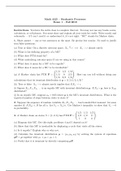#### Stochastic Processes (Exams 1, 2.a, 2.b)

(0)
\$16.48
0x  sold

Questions and answers for exams on the stochastic process. The course is designed to introduce undergraduates to the fundamental ideas of stochastic (or random) processes. Topics to be covered are Markov chains (discrete and continuous time), renewal theory, queueing theory, Brownian motion, and stationary processes.

i x
• Bundle
•  • 3 items •
• by RajR •
i x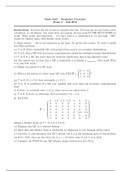#### Exam 2.a

(0)
\$7.48
0x  sold

This course is designed to introduce the undergraduate to the fundamental ideas of stochastic (or random) processes. Such processes are used in the study of mathematical models where there are elements of uncertainty and hence probabilistic quantities are introduced into the model. These models are found in fields such as the analysis of algorithms, the theory of queues, the pricing of stock options, financial mathematics, econometrics, linear programming, and biomathematics. The course will cov...

i x
•  Bundle
• Exam
•  • 5 pages •
• by RajR •
Quick View
i x
•
•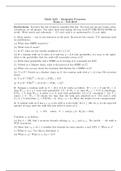#### Exam 2.b

(0)
\$7.48
0x  sold

This course is designed to introduce the undergraduate to the fundamental ideas of stochastic (or random) processes. Such processes are used in the study of mathematical models where there are elements of uncertainty and hence probabilistic quantities are introduced into the model. These models are found in fields such as the analysis of algorithms, the theory of queues, the pricing of stock options, financial mathematics, econometrics, linear programming, and biomathematics. The course will cov...

i x
•  Bundle
• Exam
•  • 3 pages •
• by RajR •
Quick View
i x
•
•#### Exam 1

(0)
\$8.48
0x  sold

This course is designed to introduce the undergraduate to the fundamental ideas of stochastic (or random) processes. Such processes are used in the study of mathematical models where there are elements of uncertainty and hence probabilistic quantities are introduced into the model. These models are found in fields such as the analysis of algorithms, the theory of queues, the pricing of stock options, financial mathematics, econometrics, linear programming, and biomathematics. The course will cov...

i x
•  Bundle
• Exam
•  • 5 pages •
• by RajR •
Quick View
i x
•
•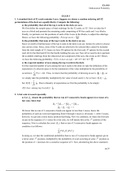#### Probability Exams (1- 3)

(0)
\$20.48
0x  sold

Probability exams testing basic theorems of probability, permutations and combinations, binomial and multinomial theorems, random variables with densities, sequences of independent identically distributed random variables, method of moments, the moment-generating function, central limit theorem, and standard-type probability distributions.

i x
• Bundle
•  • 3 items •
• by RajR •
i x#### Final Exam

(0)
\$10.48
0x  sold

This course is an introduction to the theory of probability. Topics to be included are basic theorems of probability, permutations and combinations, binomial and multinomial theorems, random variables with densities, sequences of independent identically distributed random variables, method of moments, the moment-generating function, central limit theorem, and standard-type probability distributions.

i x
•  Bundle
• Exam
•  • 12 pages •
• by RajR •
Quick View
i x
•
•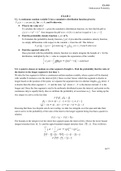#### Exam 2

(0)
\$7.48
0x  sold

This course is an introduction to the theory of probability. Topics to be included are basic theorems of probability, permutations and combinations, binomial and multinomial theorems, random variables with densities, sequences of independent identically distributed random variables, method of moments, the moment-generating function, central limit theorem, and standard-type probability distributions.

i x
•  Bundle
• Exam
•  • 10 pages •
• by RajR •
Quick View
i x
•
•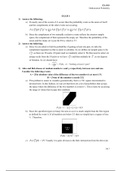#### Exam 1

(0)
\$8.48
0x  sold

This course is an introduction to the theory of probability. Topics to be included are basic theorems of probability, permutations and combinations, binomial and multinomial theorems, random variables with densities, sequences of independent identically distributed random variables, method of moments, the moment-generating function, central limit theorem, and standard-type probability distributions

i x
•  Bundle
• Exam
•  • 15 pages •
• by RajR •
Quick View
i x
•
•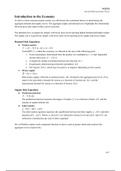(0)
\$10.48
0x  sold

Advanced topics in macro-economics with an emphasis on the theoretical understanding and the applications to forecasting cyclical and dynamic movements in the economy. The course covers calculus-based derivations of basic equilibrium models for the aggregate supply and demand curves, as well as deconstructing the primary drivers of monetary and fiscal policy with respect to the equilibrium model. Coverage includes discussion on the following topics at great length: - Product market equation...

i x
•  Book
• Class notes
•  • 28 pages •
• by RajR •